Register for Maths, Science, English, Reasoning Olympiad Exams Register here | Book Free Demo for Live Olympiad Classes here | Check Olympiad Exam Dates here | Buy Practice Papers for IMO, IOM, HEO, IOEL etc here | Login here to participate in all India free mock test on every Saturday

# Measurement of length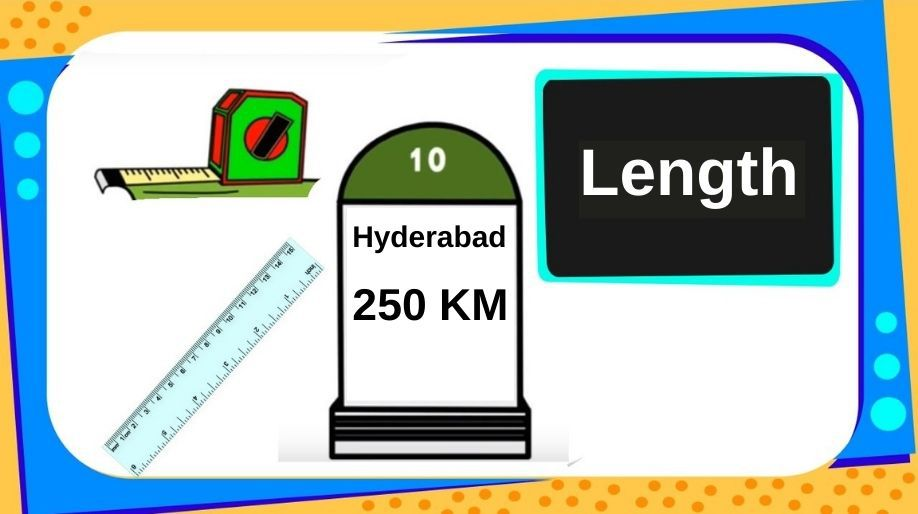• What is length? Length is the longest extent of anything.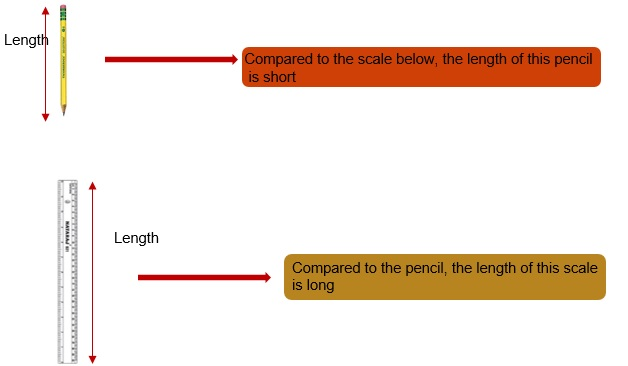• Lengths are measured in metres, centimetres, decametres and so on.

# Converting metres into centimetres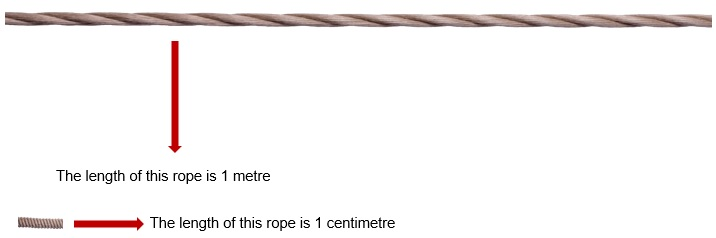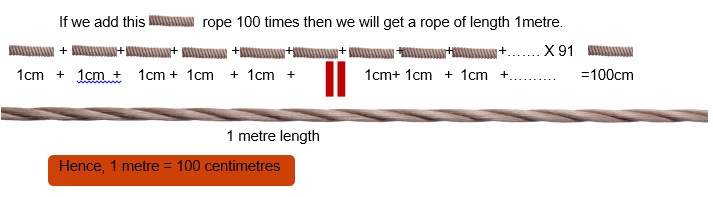• Now that you know 1 metre = 100 centimetres, can you say
• 2 metres = __________ cm
• 5 metres = __________ cm
• 13 metres = __________ cm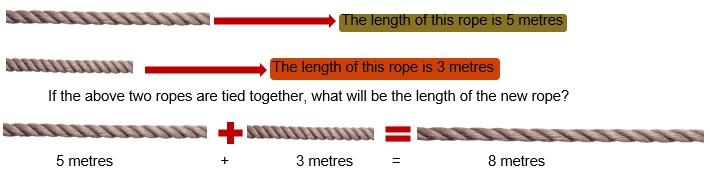# Subtraction of length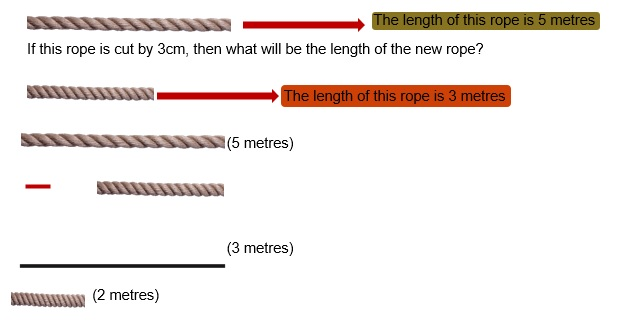# Practice these questions

Q1) Estimate the height of each picture in centimetres.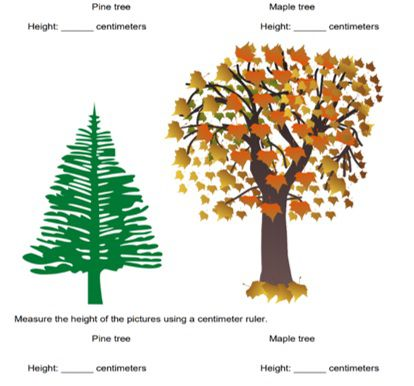Q2) Measure the height of the picture using a centimetre ruler.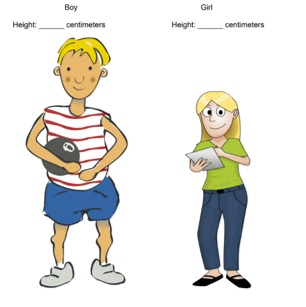• Who is taller? ______________
• By how much? ________________

Q3) Fill in the proper unit. Given: 1 metre = 100 centimetres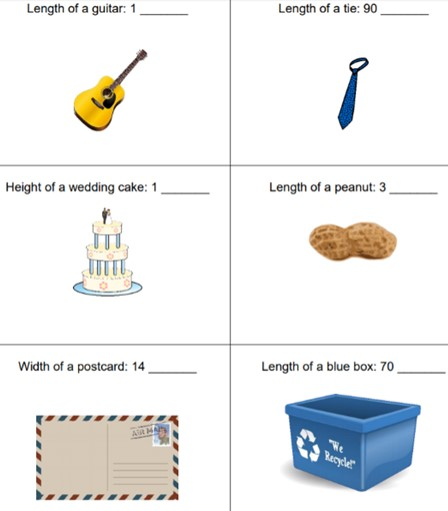## Quiz for Measurement of length

 Q.1 If one box is 1 cm, what is the length of the green line?a) 30m b) 3m c) 0.3cm d) 3cm
 Q.2 Length of each ruler is 1 m, calculate the length of the rectangle in cm.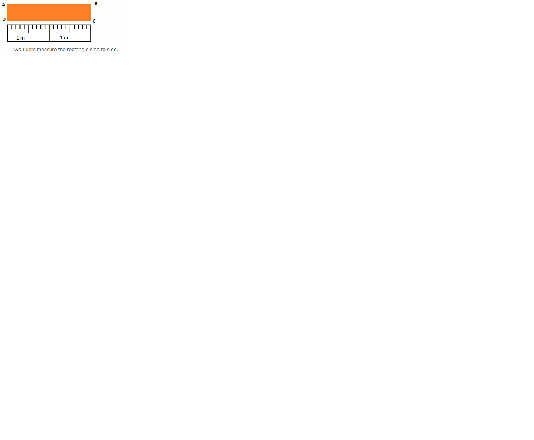a) 200cm b) 100cm c) 2m d) 4m
 Q.3 If one box is 4 cm, calculate the length of the line.a) 12 cm b) 24 m c) 24 cm d) 12 m
 Q.4 If each big division is 1 cm, give the length of the rectangle in mm.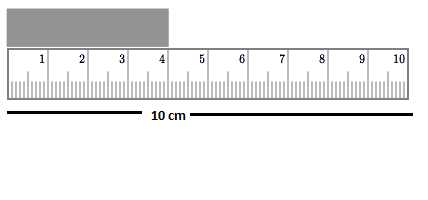a) 4m b) 40mm c) 4cm d) 400mm
 Q.5 Calculate the width of the house if each big division is one 1m. Convert your answer in cm.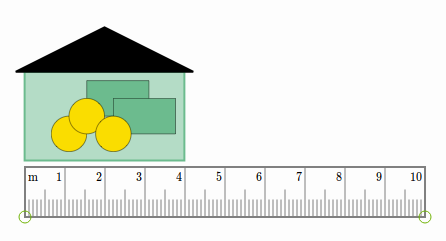a) 4m b) 40cm c) 400cm d) 300cm
 Q.6 If one box is 50 cm, how tall is the green wall? Give your answer in metres.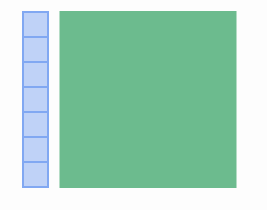a) 3m b) 5m c) 3.5m d) 4.5m
 Q.7 The scale of the map is 1cm = 15 km. What is the difference between A and B in km?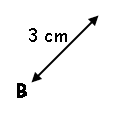a) 30km b) 3km c) 60km d) 45km
 Q.8 If each box is 30cm, calculate the length of the red rectangle in metres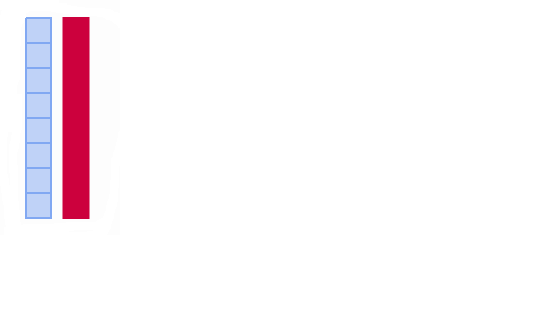a) 240m b) 240cm c) 24m d) 2.4m
 Q.9 What is the reading in the tape measure?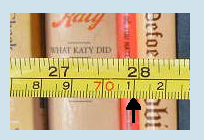a) 71 inches b) 71 cm c) 71 m d) 71mm
 Q.10 The scale of the map is 1 cm = 5 km. How many km is from B to C?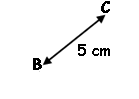a) 25km b) 5km c) 30km d) 35km